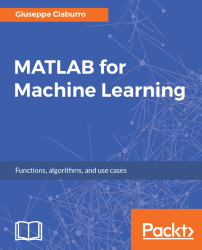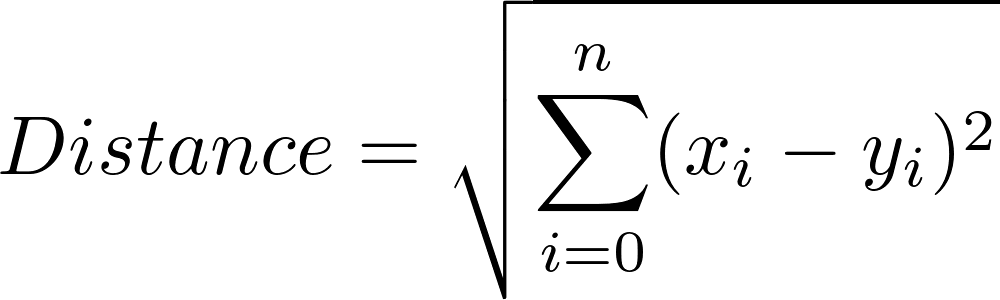•#### MATLAB for Machine Learning#### Overview of this book

MATLAB is the language of choice for many researchers and mathematics experts for machine learning. This book will help you build a foundation in machine learning using MATLAB for beginners. You’ll start by getting your system ready with t he MATLAB environment for machine learning and you’ll see how to easily interact with the Matlab workspace. We’ll then move on to data cleansing, mining and analyzing various data types in machine learning and you’ll see how to display data values on a plot. Next, you’ll get to know about the different types of regression techniques and how to apply them to your data using the MATLAB functions. You’ll understand the basic concepts of neural networks and perform data fitting, pattern recognition, and clustering analysis. Finally, you’ll explore feature selection and extraction techniques for dimensionality reduction for performance improvement. At the end of the book, you will learn to put it all together into real-world cases covering major machine learning algorithms and be comfortable in performing machine learning with MATLAB.
Title PageCreditsForewordwww.PacktPub.comCustomer FeedbackPrefaceFree Chapter
Getting Started with MATLAB Machine LearningImporting and Organizing Data in MATLABFrom Data to Knowledge DiscoveryFinding Relationships between Variables - Regression TechniquesPattern Recognition through Classification AlgorithmsIdentifying Groups of Data Using Clustering MethodsSimulation of Human Thinking - Artificial Neural NetworksImproving the Performance of the Machine Learning Model - Dimensionality ReductionMachine Learning in Practice## Find similarities using nearest neighbor classifiers

In the classification analysis, the objective is to verify the existence of differences between classes according to the variables considered. This leads to the formulation of a model that can assign each sample to the class to which it belongs. If the model is obtained from a set whose classes are known (training set), the predictive power of the model itself can be verified by using another set of data (evaluation set) also with a known class. Those samples are classified according to the previously elaborated model.

Among the different types of existing classifiers, we also find the nearest neighbor, which identifies the class of belonging to a tested sample based on the distance of this from stored and classified objects. In most cases, the distance is defined as Euclidean distance between two points, calculated according to the following formula:On a bidimensional plane, the Euclidean distance represents the minimum distance between...